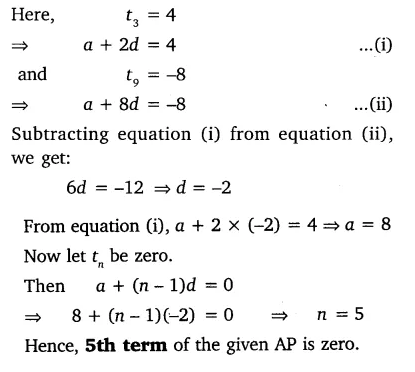# If the 3rd and the 9th term of an AP are 4 and -8 respectively, which term of this AP is zero?

If the 3rd and the 9th term of an AP are 4 and -8 respectively, which term of this AP is zero?